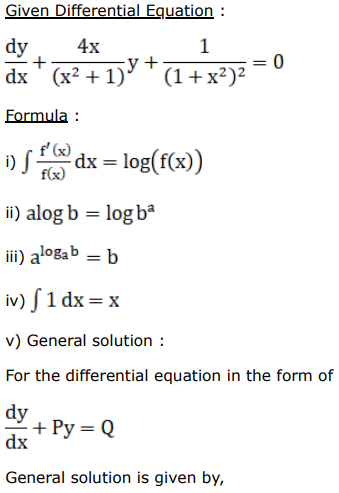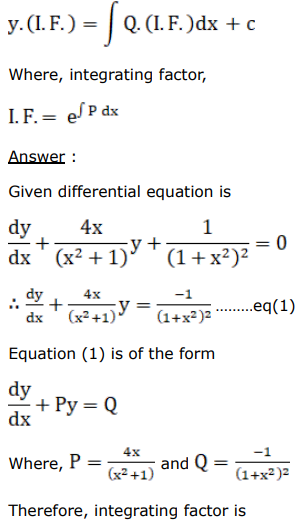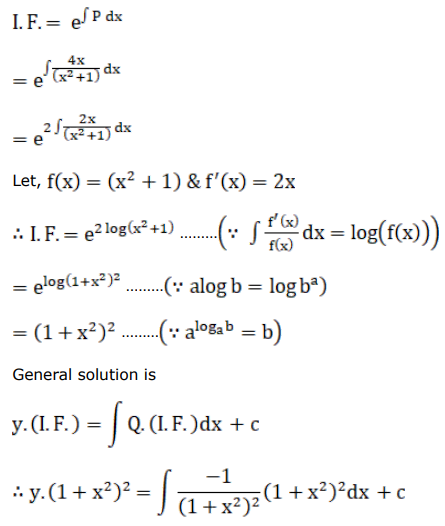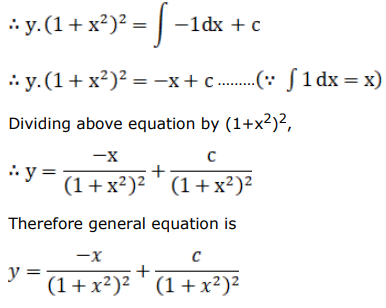# Find the general solution for each of the following differential equations.

Question:

Find the general solution for each of the following differential equations.

$\frac{d y}{d x}+\frac{4 x}{\left(x^{2}+1\right)} y+\frac{1}{\left(x^{2}+1\right)^{2}}=0$

Solution: